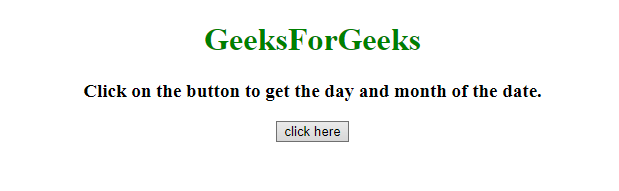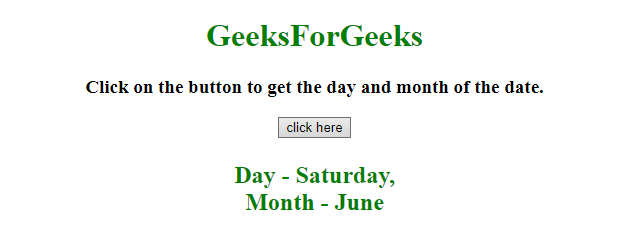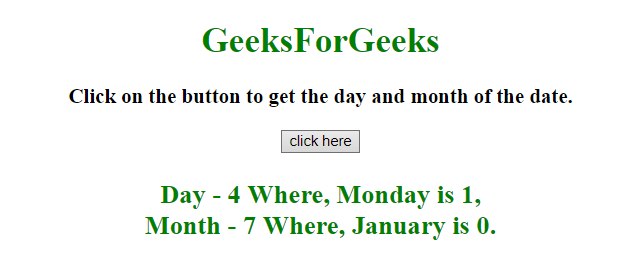# How to get the day and month of a year using JavaScript ?

Given a date and the task is to get the day and month of a year using JavaScript.

Approach:

• First get the current date by using new Date().
• Use currentDate.getDay() to get the current day in number format, Map it to the day name.
• Use currentDate.getMonth() to get the current month in number format, Map it to the month name.

Example 1: In this example, the month and date is determined by the above approach.

 `  ` `<``html``>  ` ` `  `<``head``>  ` `    ``<``title``>  ` `        ``How to get the day and month ` `        ``of a year using JavaScript ? ` `    `` ` `  ` ` `  `<``body` `style` `= ``"text-align:center;"``>  ` `     `  `    ``<``h1` `style` `= ``"color:green;"` `>  ` `        ``GeeksForGeeks ` `    `` ` `     `  `    ``<``p` `id` `= ``"GFG_UP"` `style` `= ` `        ``"font-size: 19px; font-weight: bold;"``> ` `    `` ` `     `  `    ``<``button` `onclick` `= ``"GFG_Fun()"``> ` `        ``click here ` `    `` ` `     `  `    ``<``p` `id` `= ``"GFG_DOWN"` `style` `= ` `        ``"color: green; font-size: 24px; font-weight: bold;"``> ` `    `` ` `     `  `    ``<``script``> ` `        ``var el_up = document.getElementById("GFG_UP"); ` `        ``var el_down = document.getElementById("GFG_DOWN"); ` `         `  `        ``el_up.innerHTML = "Click on the button to get " ` `                ``+ "the day and month of the date."; ` `         `  `        ``var Days = ['Sunday', 'Monday', 'Tuesday',  ` `                ``'Wednesday', 'Thursday', 'Friday', 'Saturday']; ` ` `  `        ``var Months = ['January', 'February', 'March', 'April',  ` `                ``'May', 'June', 'July', 'August', 'September', ` `                ``'October', 'November', 'December']; ` `         `  `        ``var currentDay = new Date(); ` `         `  `        ``// Get the current day name ` `        ``var day = Days[currentDay.getDay()]; ` `         `  `        ``// Get the current month name ` `        ``var month = Months[currentDay.getMonth()];  ` `         `  `        ``function GFG_Fun() { ` `            ``el_down.innerHTML = "Day - " + day ` `                    ``+ ",<``br``> Month - " + month; ` `        ``} ` `    ``  ` `  ` ` `  ` `

Output:

• Before clicking on the button:• After clicking on the button:Example 2: This is same example but with a different approach. In this example, the month and date is determined by the above approach.

 `  ` `<``html``>  ` ` `  `<``head``>  ` `    ``<``title``>  ` `        ``How to get the day and month of ` `        ``a year using JavaScript ? ` `    `` ` `  ` ` `  `<``body` `style` `= ``"text-align:center;"``>  ` `     `  `    ``<``h1` `style` `= ``"color:green;"` `>  ` `        ``GeeksForGeeks ` `    `` ` `     `  `    ``<``p` `id` `= ``"GFG_UP"` `style` `= ` `        ``"font-size: 19px; font-weight: bold;"``> ` `    `` ` `     `  `    ``<``button` `onclick` `= ``"GFG_Fun()"``> ` `        ``click here ` `    `` ` `     `  `    ``<``p` `id` `= ``"GFG_DOWN"` `style` `= ` `        ``"color: green; font-size: 24px; font-weight: bold;"``> ` `    `` ` `     `  `    ``<``script``> ` `        ``var el_up = document.getElementById("GFG_UP"); ` `        ``var el_down = document.getElementById("GFG_DOWN"); ` `     `  `        ``el_up.innerHTML = "Click on the button to get the " ` `                    ``+ "day and month of the date."; ` `         `  `        ``var currentDay = new Date(); ` `         `  `        ``// Get the current day name ` `        ``var day = currentDay.getDay();  ` `         `  `        ``// Getting the current month name ` `        ``var month = currentDay.getMonth();  ` `         `  `        ``function GFG_Fun() { ` `            ``el_down.innerHTML = "Day - " + day +  ` `                    ``" Where, Monday is 1,<``br``> Month - " ` `                    ``+ month +" Where, January is 0."; ` `        ``} ` `    ``  ` `  ` ` `  ` `

Output:

• Before clicking on the button:• After clicking on the button:My Personal Notes arrow_drop_upCheck out this Author's contributed articles.

If you like GeeksforGeeks and would like to contribute, you can also write an article using contribute.geeksforgeeks.org or mail your article to contribute@geeksforgeeks.org. See your article appearing on the GeeksforGeeks main page and help other Geeks.

Please Improve this article if you find anything incorrect by clicking on the "Improve Article" button below.